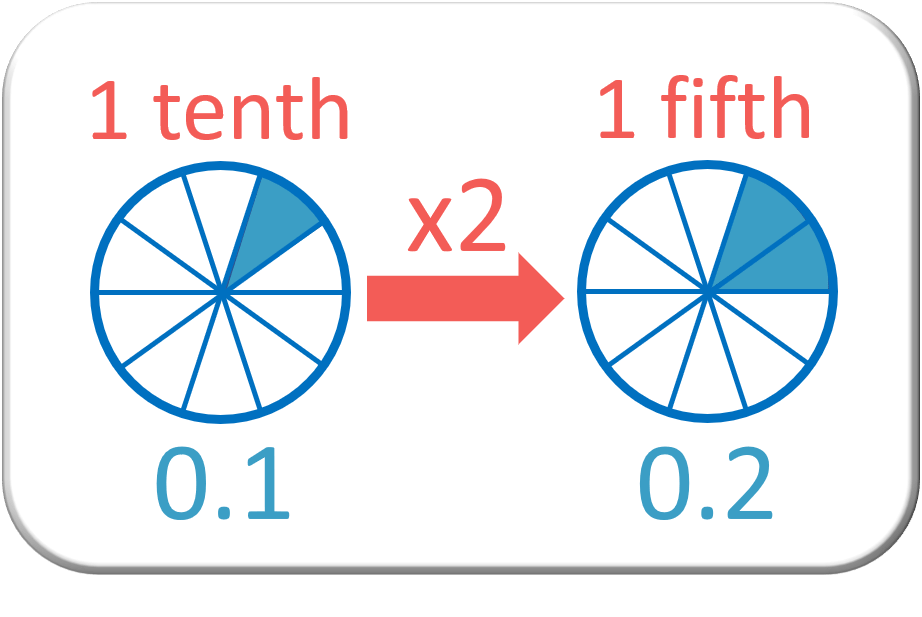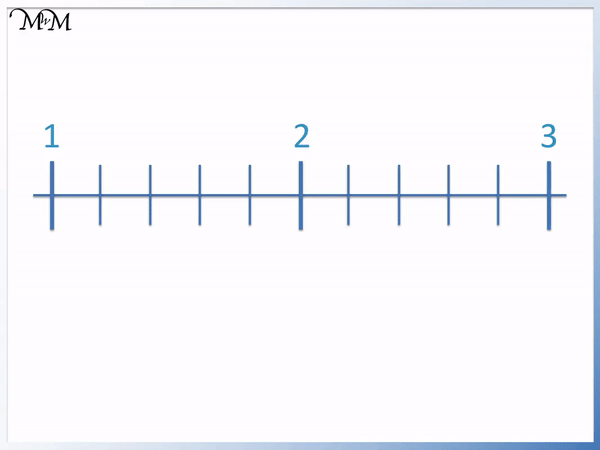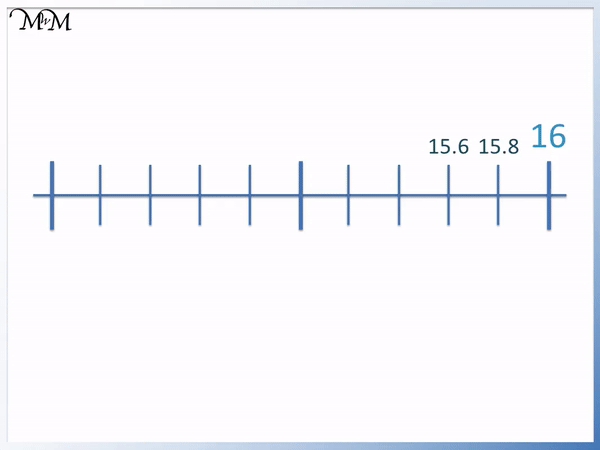# Decimals on a Number Line: Counting in Fifths

Decimals on a Number Line: Counting in Fifths• Here is a number line between zero and one.
• There are 4 lines dividing the line into 5 equal parts.
• One whole divided into five equal parts is   1 / 5  .
•   1 / 5   as a decimal is 0.2.
• At each new line, we are adding 0.2 or one fifth.
• Counting in fifths is the same as counting in jumps of 0.2. The digit after the decimal point increases by 2 each time.
• We have 0.2, 0. 4, 0.6, 0.8 and 1.0.
• This is like the two times table but ten times smaller.

• Five tenths is equal to one whole.
A fifth written as a decimal is 0.2.

Counting in fifths is counting up in 0.2.• There are 5 equal parts between each number and so we are counting up in fifths.
• Moving from one line to the next we are counting up in jumps of 0.2.
• We can count on in jumps of 0.2 from 25: 25.2 and then 25.4.
• We can count on in jumps of 0.2 from 26: 26.2, 26.4, 26.6 and then 26.8.
• The two decimal numbers indicated by the arrows are 25.4 and 26.8# Counting in Fifths with Decimals on a Number Line

In this lesson, we will look at placing missing decimals on a number line when counting in fifths.

One fifth as a decimal is 0.2

One fifth is double the size of one tenth.One tenth is 0.1 and so, one fifth is 0.2, which is double the size of 0.1.In the animation below, we have a number line ranging from zero to one.

To get from zero to one, there are ten steps. This means that we will be counting up in tenths, or 0.1s.The first line on the scale corresponds to an increment of 0.1, which is the same as one tenth.

The second line is 0.2, which is two tenths.

The third line is 0.3, which is three tenths.

The fourth line is 0.4, which is four tenths.

The fifth line is 0.5, which is five tenths. This thicker line indicates that it is the halfway point between 0 and 1.

The sixth line is 0.6, which is six tenths.

The seventh line is 0.7, which is seven tenths.

The eighth line is 0.8, which is eight tenths.

The ninth line is 0.9, which is nine tenths.

The tenth line is 1, which is the same as ten tenths, or one whole.

We know that 5 lots of 0.2 makes a whole, or 1.In the animation below, we have a scale ranging from zero to one. However, this time, to get from zero to one, we have five steps.The scale is divided into five parts, or fifths. This means that we will be counting up in fifths, or 0.2s.

This is because, instead of taking ten steps, we are taking five steps. So, we need to cover twice the distance in each step. Therefore, instead of counting in 0.1s, we need to count in 0.2s.

The first mark on the scale is 0.2, which is the same as one fifth.

The second mark is 0.4, which is two fifths.

The third mark is 0.6, which is three fifths.

The fourth mark is 0.8, which is four fifths.

The fifth mark is 1, which is the same as five fifths, or one whole.

Below, we have a scale ranging from one to two and from two to three.

To get from one to two, there are five steps and to get from two to three there are five steps.

We are asked to label the values on the number line that are indicated by the arrows.Because there are five steps from one to two and from two to three, we are counting in fifths, or 0.2s.

So, we can begin by counting up from one. We have 1.2, 1.4 and 1.6, which is our first answer.

Next, we can count on from two. We have 2.2 and 2.4, which is our second answer.

In the following example, the scale ranges from 25 to 27.The thicker line in the centre is the halfway point. The number that is halfway between 25 and 27 is 26.

To get from 25 to 26, there are five steps. So, we are counting in 0.2s.

Starting from 25, we have 25.2 and 25.4, which is our first answer.

Starting from 26, we have 26.2, 26.4, 26.6 and 26.8, which is our second answer.

We could have also found the second answer by counting back in 0.2s from 27.

On the scale below, we are given the numbers 15.6, 15.8 and 16.

To get from 15.6 to 15.8 and from 15.8 to 16, we add 0.2. This tells us that we are counting in 0.2s.We can begin by counting back from 15.6. we have 15.4 and 15.2, which is our first answer.

Continuing, we have 15, 14.8 and 14.6, which is our second answer.

Continuing to count back from 14.6, we have 14.4, 14.2 and 14, which is our third answer.Now try our lesson on Converting Decimals to Fractions where we learn how to write a decimal as a fraction.error: Content is protected !!